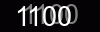# How to load a PDF document in C# and VB.Net

``````
``````
The dc object represents a document loaded into memory. The file format is detected automatically from the file extension: ".pdf" .

After loading you'll get the document presented as the Tree Of Objects, where the root node is DocumentCore class.

``````
``````
``````
// Assume that we already have a PDF document as bytes array.
byte[] pdfBytes = null;
// pdfBytes = ...

DocumentCore dc = null;
using (MemoryStream pdfStream = new MemoryStream(fileBytes))
{
// Specifying PdfLoadOptions we explicitly set that a loadable document is PDF.
{
// 'false' - means to load vector graphics as is. Don't transform it to raster images.
RasterizeVectorGraphics = false,

// The PDF format doesn't have real tables, in fact it's a set of orthogonal graphic lines.
// In case of 'true' the component will detect and recreate tables from graphic lines.
DetectTables = false,

// 'true' - Load embedded fonts from PDF document, even if the font with the same name is installed in your System.
PreserveEmbeddedFonts = false,

// Load only the 1st page from the document.
PageIndex = 0,
PageCount = 1
};

// Load a PDF document from the MemoryStream.
}
// Here we can do with our document 'dc' anything we need.``````

## Complete code

``````using System;
using System.IO;
using SautinSoft.Document;

namespace Example
{
class Program
{

static void Main(string[] args)
{
}

/// <summary>
/// Loads a PDF document into DocumentCore (dc) from a file.
/// </summary>
/// <remarks>
/// </remarks>
{
string filePath = @"..\..\example.pdf";

// The file format is detected automatically from the file extension: ".pdf".
// But as shown in the example below, we can specify PdfLoadOptions as 2nd parameter
// to explicitly set that a loadable document has PDF format.

if (dc != null)
}

/// <summary>
/// Loads a PDF document into DocumentCore (dc) from a MemoryStream.
/// </summary>
/// <remarks>
/// </remarks>
{
// Assume that we already have a PDF document as bytes array.

DocumentCore dc = null;

// Create a MemoryStream
using (MemoryStream pdfStream = new MemoryStream(fileBytes))
{
// Specifying PdfLoadOptions we explicitly set that a loadable document is PDF.
{
// 'false' - means to load vector graphics as is. Don't transform it to raster images.
RasterizeVectorGraphics = false,

// The PDF format doesn't have real tables, in fact it's a set of orthogonal graphic lines.
// In case of 'true' the component will detect and recreate tables from graphic lines.
DetectTables = false,

// 'true' - Load embedded fonts from PDF document, even if the font with the same name is installed in your System.
PreserveEmbeddedFonts = false,

// Load only the 1st page from the document.
PageIndex = 0,
PageCount = 1
};

// Load a PDF document from the MemoryStream.
}
if (dc != null)
}
}
}
``````
```
```Imports System
Imports System.IO
Imports SautinSoft.Document

Module Sample
Sub Main()
End Sub

''' <summary>
''' Loads a PDF document into DocumentCore (dc) from a file.
''' </summary>
''' <remarks>
''' </remarks>
Dim filePath As String = "..\example.pdf"

' The file format is detected automatically from the file extension: ".pdf".
' But as shown in the example below, we can specify PdfLoadOptions as 2nd parameter
' to explicitly set that a loadable document has PDF format.
Dim dc As DocumentCore = DocumentCore.Load(filePath)

If dc IsNot Nothing Then
End If
End Sub

''' <summary>
''' Loads a PDF document into DocumentCore (dc) from a MemoryStream.
''' </summary>
''' <remarks>
''' </remarks>
' Assume that we already have a PDF document as bytes array.
Dim fileBytes() As Byte = File.ReadAllBytes("..\example.pdf")

Dim dc As DocumentCore = Nothing

' Create a MemoryStream
Using pdfStream As New MemoryStream(fileBytes)

' Specifying PdfLoadOptions we explicitly set that a loadable document is PDF.
Dim pdfLO As New PdfLoadOptions() With {
.RasterizeVectorGraphics = False,
.DetectTables = False,
.PreserveEmbeddedFonts = False,
.PageIndex = 0,
.PageCount = 1
}

' RasterizeVectorGraphics = False
' This means to load vector graphics as is. Don't transform it to raster images.

' DetectTables = False
' This means don't detect tables.
' The PDF format doesn't have real tables, in fact it's a set of orthogonal graphic lines.
' Set it to 'True' and the component will detect and recreate tables from graphic lines.

'PreserveEmbeddedFonts = False
' True - Means to load embedded fonts from PDF document,
' even if the font with the same name is installed in your System.

' Load a PDF document from the MemoryStream.
End Using
If dc IsNot Nothing Then